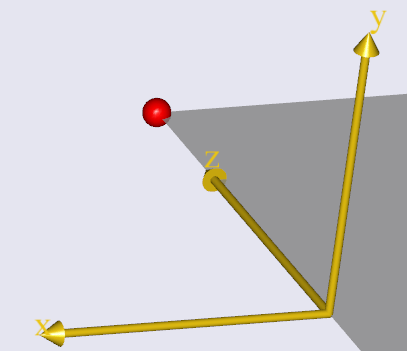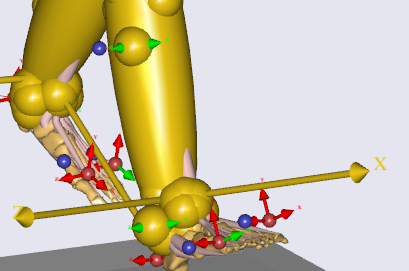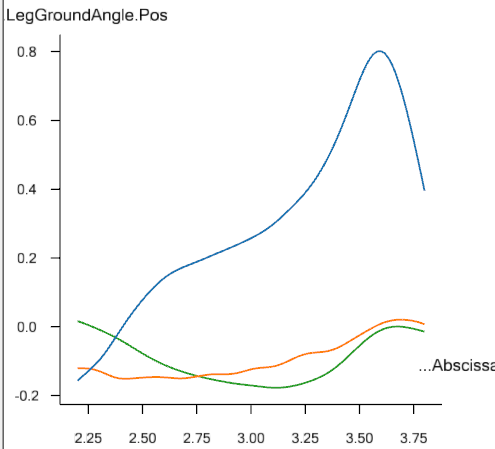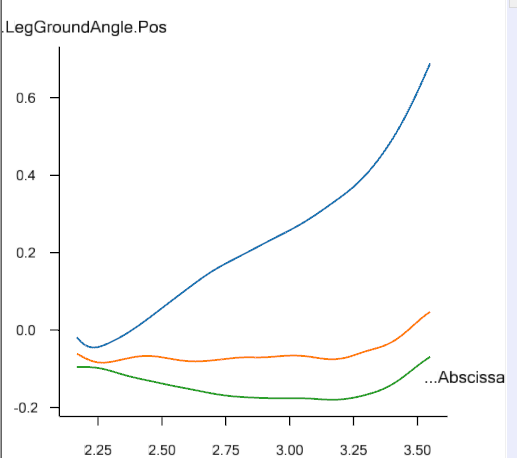# Angle of shank to ground

Hi,
Taking LowerExtremity.any as an example, I want to get the angle between the shank and the ground. I obtained it in the following way, but the result seems to have some problems, and the relationship with the movement does not match.
First, I defined a new coordinate system in the world coordinate system and made it consistent with the shank coordinate system.

``````AnyFixedRefFrame GlobalRef ={
Origin={0,0,0};
AnyDrawRefFrame drw={ScaleXYZ=0.2*{1,1,1};};
AnyRefNode GL ={
sRel = {0,0.3,0};
ARel = {{-1,0,0},{0,0,1},{0,1,0}};
AnyDrawRefFrame drw={ScaleXYZ=0.2*{1,1,1};};
};

``````

Here are the coordinates of the GL point,This is the coordinates of the shank,Then, I defined the angle between the lower leg and the ground in the following way in the output of the result. The ShankJoint is a point I defined on the mid-section of the shank.

``````AnyFolder LegGroundAngle = {

AnyKinMeasureOrg LegGroundAngle = {
AnyKinRotational LegGround ={
AnyRefNode &Shank2Node = Main.HumanModel.BodyModel.Right.Leg.Seg.Shank.ShankJoint;
AnyRefNode &Global = Main.EnvironmentModel.GlobalRef.GL;
Type=RotAxesAngles;
};
};
};

``````

In this way, three curves are obtained, but I find that these three curves and the movement trend do not seem to be aligned, and I cannot obtain the angle information of the calf and the ground that I want. Could you please tell me how to get what I want?I would be very grateful to hear from you.

Best Regards.
Xu

I think Pos is the angle between shank's y-axis coordinate and GL's y-axis coordinate, that is, the angle between shank and the plane perpendicular to the ground. But the trend of this curve does not match the angle of movement.

Hi,
I would like to add some more detailed information to you. Below is a short video. What I want to get is the angle between the shank of the right leg and the ground. From the video, we can see that the angle between the y-axis of the shank and the y-axis of the GL coordinate system is gradually increasing. But the obtained Pos, that is, the orange line in the figure below is indeed gradually decreasing, which cannot match the movement situation.
video.zip (1.2 MB)Best regards.
Xu

Hi Xu,

Sorry for the delayed response.

I don't exactly understand the orientation of the GL coordinate system in relation to the shank coordinate system but as you mentioned they are consistent, then I will work with this assumption.

In case of the shank, the y axis is the vertical (proximal-distal) and the z axis is the medial lateral axis. So, if you want to measure the angle between y axis of the shank and the y axis of the GL frame, you should be looking at the rotation about the z-axis, which is in this case Pos (the blue line). I think the blue curve looks alright and gives exactly what you are looking for.

Best regards,
Dave